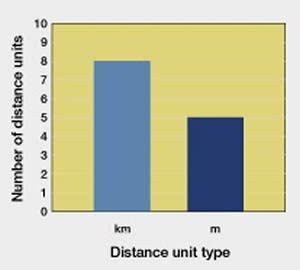# Difference between Kilometers and Miles

##### Key Difference: Kilometer and mile are two different units of measurement of distances. One kilometer is equivalent to 0.621 miles.Kilometer is the metric unit of length which is used for measuring long distances. The smallest unit among the metric units used for measuring length is millimeter. Centimeter comes next which is equivalent to 10 millimeters. After this, meter is defined which is equivalent to 100 centimeters. Finally, one kilometer is generally defined in terms of meters as it is equivalent to 1000 meters. The short form of kilometer is km.

Miles is another measure of distance. It is considered to be a traditional unit for measurement. However, in the countries like U.S., etc., it is still used as the main unit for measurement of distance. It is originally a Roman unit which was earlier defined as 1000 paces of a Roman legion. The England distance system set the length of the mile at 8 furlongs in 1573. This length of a furlong was set by the early Tudor rulers and they defined is as equivalent to 220 yards.

This mile is equivalent to 1760 yards. It is also equal to 1.609 kilometers. Nautical mile is used for measuring distances at sea. It is equivalent to 1852 meters.

Therefore, kilometer and mile are two different units for measuring distances. One kilometer is equivalent to 1000 meters, whereas one mile is equal to 1760 yards or 1.609 kilometers.

Comparison between Kilometers and Miles:

 Kilometers Miles Definition A unit based on the metric system; used for measuring distances Another unit for measuring distances Value It is equivalent to 1000 metres It is equivalent to 1760 yards I km/mile is equivalent to 0.621 miles 1.609 kilometers Yards Feet Metres Inches Centimeters 1 km = 1093.613 yd 1 km = 3280.84 ft 1 km = 1000 m 1 km = 39,370 in 1 km = 100000 cm 1 mile = 1,760 yd 1 mile = 5,280 ft 1 mile = 1609.344 m 1 mile = 63,360 in 1 mile = 160934.4 cm Abbreviation Km Mi

Image Courtesy: runrichard.co.uk